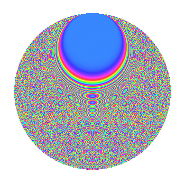# Properties

 Label 2541.2.bfLevel 2541 Weight 2 Character orbit bf Rep. character $$\chi_{2541}(76,\cdot)$$ Character field $$\Q(\zeta_{22})$$ Dimension 1760 Sturm bound 704

# Related objects

## Defining parameters

 Level: $$N$$ = $$2541 = 3 \cdot 7 \cdot 11^{2}$$ Weight: $$k$$ = $$2$$ Character orbit: $$[\chi]$$ = 2541.bf (of order $$22$$ and degree $$10$$) Character conductor: $$\operatorname{cond}(\chi)$$ = $$847$$ Character field: $$\Q(\zeta_{22})$$ Sturm bound: $$704$$

## Dimensions

The following table gives the dimensions of various subspaces of $$M_{2}(2541, [\chi])$$.

Total New Old
Modular forms 3560 1760 1800
Cusp forms 3480 1760 1720
Eisenstein series 80 0 80

## Trace form

 $$1760q + 172q^{4} - 1760q^{9} + O(q^{10})$$ $$1760q + 172q^{4} - 1760q^{9} + 28q^{11} + 28q^{14} + 8q^{15} - 172q^{16} - 24q^{22} - 68q^{23} + 184q^{25} - 172q^{36} - 132q^{37} - 16q^{42} + 54q^{44} + 52q^{49} + 232q^{53} + 48q^{56} - 14q^{58} - 24q^{60} + 72q^{64} - 64q^{67} - 52q^{70} + 16q^{71} - 24q^{78} + 88q^{79} + 1760q^{81} - 88q^{85} - 16q^{86} - 44q^{88} - 82q^{91} - 312q^{92} + 24q^{93} - 28q^{99} + O(q^{100})$$

## Decomposition of $$S_{2}^{\mathrm{new}}(2541, [\chi])$$ into newform subspaces

The newforms in this space have not yet been added to the LMFDB.

## Decomposition of $$S_{2}^{\mathrm{old}}(2541, [\chi])$$ into lower level spaces

$$S_{2}^{\mathrm{old}}(2541, [\chi]) \cong$$ $$S_{2}^{\mathrm{new}}(847, [\chi])$$$$^{\oplus 2}$$

## Hecke Characteristic Polynomials

There are no characteristic polynomials of Hecke operators in the database